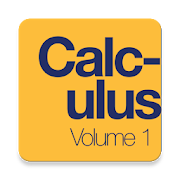# Calculus Volume 1 Textbook, Test BankEveryone
26
Calculus is designed for the typical two- or three-semester general calculus course, incorporating innovative features to enhance student learning.
The book guides students through the core concepts of calculus and helps them understand how those concepts apply to their lives and the world around them.
Due to the comprehensive nature of the material, we are offering the book in three volumes for flexibility and efficiency.
Volume 1 covers functions, limits, derivatives, and integration.

* Complete Textbook by OpenStax
* Multiple Choices Questions (MCQ)
* Essay Questions Flash Cards
* Key-Terms Flash Cards

https://www.quizover.com

1. Functions and Graphs
Introduction
1.1. Review of Functions
1.2. Basic Classes of Functions
1.3. Trigonometric Functions
1.4. Inverse Functions
1.5. Exponential and Logarithmic Functions
2. Limits
Introduction
2.1. A Preview of Calculus
2.2. The Limit of a Function
2.3. The Limit Laws
2.4. Continuity
2.5. The Precise Definition of a Limit
3. Derivatives
Introduction
3.1. Defining the Derivative
3.2. The Derivative as a Function
3.3. Differentiation Rules
3.4. Derivatives as Rates of Change
3.5. Derivatives of Trigonometric Functions
3.6. The Chain Rule
3.7. Derivatives of Inverse Functions
3.8. Implicit Differentiation
3.9. Derivatives of Exponential and Logarithmic Functions
4. Applications of Derivatives
Introduction
4.1. Related Rates
4.2. Linear Approximations and Differentials
4.3. Maxima and Minima
4.4. The Mean Value Theorem
4.5. Derivatives and the Shape of a Graph
4.6. Limits at Infinity and Asymptotes
4.7. Applied Optimization Problems
4.8. L’Hôpital’s Rule
4.9. Newton’s Method
4.10. Antiderivatives
5. Integration
Introduction
5.1. Approximating Areas
5.2. The Definite Integral
5.3. The Fundamental Theorem of Calculus
5.4. Integration Formulas and the Net Change Theorem
5.5. Substitution
5.6. Integrals Involving Exponential and Logarithmic Functions
5.7. Integrals Resulting in Inverse Trigonometric Functions
6. Applications of Integration
Introduction
6.1. Areas between Curves
6.2. Determining Volumes by Slicing
6.3. Volumes of Revolution: Cylindrical Shells
6.4. Arc Length of a Curve and Surface Area
6.5. Physical Applications
6.6. Moments and Centers of Mass
6.7. Integrals, Exponential Functions, and Logarithms
6.8. Exponential Growth and Decay
6.9. Calculus of the Hyperbolic Functions
Table of Integrals
Table of Derivatives
Review of Pre-Calculus
Collapse

## Reviews

Review Policy
4.5
26 total
5
4
3
2
1

Updated
March 20, 2018
Size
5.5M
Installs
10,000+
Current Version
2.1.1
Requires Android
4.4 and up
Content Rating
Everyone
Interactive Elements
Users Interact
Permissions
Offered By
QuizOver.com